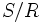Permutation kernel

Suppose$G$ is a finite group,$R = \operatorname{Rad}(G)$ is its solvable radical, and$S = \operatorname{Soc}^*(G)$ is its socle over solvable radical, i.e.,$S/R$ is the socle of$G/R$.$S/R$ can be expressed uniquely as a direct product of simple non-abelian groups.
The permutation kernel of$G$, denoted$\operatorname{PKer}(G)$, is the kernel of the action of$G$ on these factors induced by the action of$G$ on$S/R$ by conjugation.$\operatorname{PKer}(G)$ contains the socle over solvable radical$S$.
The permutation kernel is part of the Babai-Beals filtration of$G$.